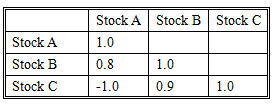### CFA Practice Question

There are 294 practice questions for this study session.

### CFA Practice Question

Stocks A, B, and C each have the same expected return and standard deviation. Given the correlation coefficients between the stocks shown in the table below, which combination of these stocks will result in the lowest-risk portfolio?A. Equal investment in stocks A and B
B. Equal investment in stocks B and C
C. Equal investment in stocks A and C
Explanation: If everything else is equal, the portfolio that has the lowest (or most negative) correlation will have the lowest risk.